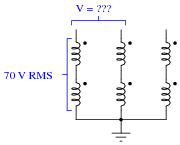# AC Generator Theory

## AC Electric Circuits

• #### Question 1

If an electric current is passed through this wire, which direction will the wire be pushed (by the interaction of the magnetic fields)?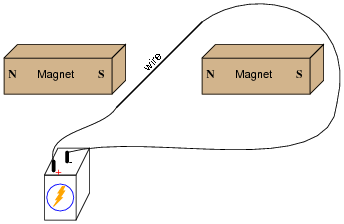Is this an example of an electric motor or an electric generator?

• #### Question 2

If this wire (between the magnet poles) is moved in an upward direction, what polarity of voltage will the meter indicate?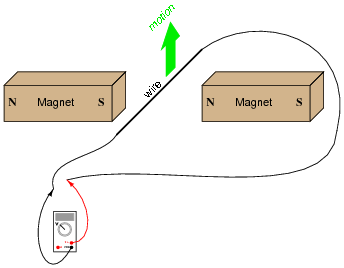Describe the factors influencing the magnitude of the voltage induced by motion, and determine whether this is an example of an electric motor or an electric generator.

• #### Question 3

If this wire (between the magnet poles) is moved in an upward direction, and the wire ends are connected to a resistive load, which way will current go through the wire?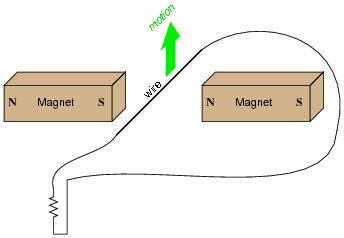We know that current moving through a wire will create a magnetic field, and that this magnetic field will produce a reaction force against the static magnetic fields coming from the two permanent magnets. Which direction will this reaction force push the current-carrying wire? How does the direction of this force relate to the direction of the wire’s motion? Does this phenomenon relate to any principle of electromagnetism you’ve learned so far?

• #### Question 4

Determine the polarity of induced voltage between the ends of this wire loop, as it is rotated between the two magnets: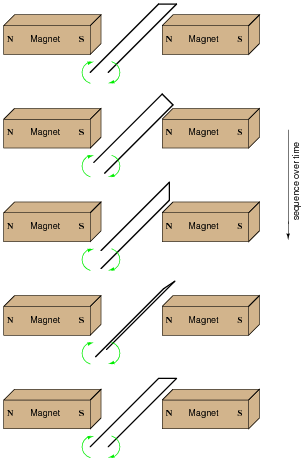• #### Question 5

Describe the nature of the voltage induced in the stationary (“stator”) windings, as the permanent magnet rotor rotates in this machine: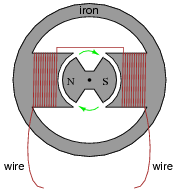What factors determine the magnitude of this voltage? According to Faraday’s Law, what factors can we alter to increase the voltage output by this generator?

Is the induced voltage AC or DC? How can you tell?

• #### Question 6

In order to make the most practical AC generator (or alternator, as it is also known), which design makes more sense: a stationary permanent magnet with a rotating wire coil, or a rotating permanent magnet with a stationary wire coil? Explain your choice.

• #### Question 7

We know that in order to induce a sinusoidal voltage in a wire coil, the magnetic flux linking the turns of wire in the coil must follow a sinusoidal path over time, phase-shifted 90o from the voltage waveform. This relationship between flux and induced voltage is expressed in Faraday’s equation v = N [(dφ)/dt]: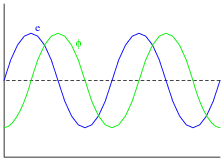Based on this fact, draw the position of the magnetic rotor in this alternator when the voltage is at one of its peaks: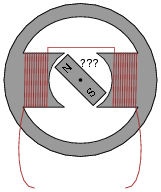• #### Question 8

If this alternator is spun at 4500 RPM (revolutions per minute), what will be the frequency of its output voltage?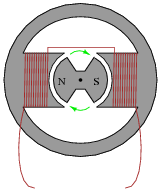Hint: how many cycles of AC are produced for every revolution of the rotor?

• #### Question 9

How fast must a 12-pole alternator spin in order to produce 60 Hz AC power? Write a mathematical equation solving for speed (S) in terms of frequency (f) and the number of poles (N).

• #### Question 10

How many poles does an alternator have if it generates 400 Hz power at a shaft speed of 6000 RPM?

• #### Question 11

Assuming that the output frequency of an alternator must remain constant (as is the case in national power systems, where the frequency of all power plants must be the same), how may its output voltage be regulated? In other words, since we do not have the luxury of increasing or decreasing its rotational speed to control voltage, since that would change the frequency, how can we coax the alternator to produce more or less voltage on demand?

Hint: automotive alternators are manufactured with this feature, though the purpose in that application is to maintain constant voltage despite changes in engine speed. In automotive electrical systems, the frequency of the alternator’s output is irrelevant because the AC is “rectified” into DC (frequency = 0 Hz) to charge the battery.

• #### Question 12

Suppose we have an alternator with two sets of windings, A and B: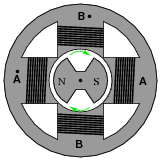Each pair of windings in each set is series-connected, so they act as just two separate windings:If one end of each winding pair were connected together at a common ground point, and each winding pair output 70 volts RMS, how much voltage would be measured between the open winding pair ends?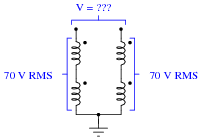• #### Question 13

Suppose we have an alternator with three sets of windings, A, B, and C: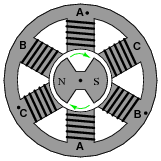Each pair of windings in each set is series-connected, so they act as just three separate windings (pay close attention to the phase-marking dots!):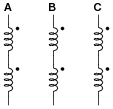If one end of each winding pair were connected together at a common ground point, and each winding pair output 70 volts RMS, how much voltage would be measured between any two open wires?